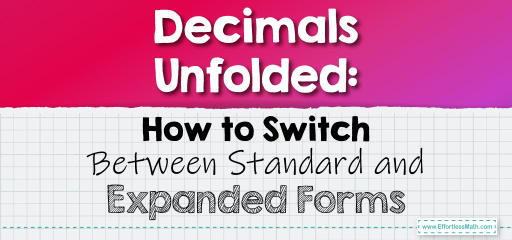# Decimals Unfolded: How to Switch Between Standard and Expanded Forms

Decimals can be represented in multiple ways, and two of the most common forms are the standard form and the expanded form. The standard form is how we typically write decimals, while the expanded form breaks down each digit's value based on its place. Let's delve into the art of converting between these two forms!## Converting Decimals Between Standard and Expanded Form

### Example 1:

Standard Form: $$3.42$$

Expanded Form: To convert this into expanded form:

– The digit $$3$$ is in the ones place: $$3 \times 1$$

– The digit $$4$$ is in the tenths place: $$4 \times 0.1$$

– The digit $$2$$ is in the hundredths place: $$2 \times 0.01$$

Combine these values: $$3 + 0.4 + 0.02$$

The expanded form of $$3.42$$ is $$3 + 0.4 + 0.02$$.

The Absolute Best Book for 5th Grade Students

### Example 2:

Standard Form: $$5.067$$

Expanded Form:

– The digit $$5$$ is in the ones place: $$5 \times 1$$

– The digit $$0$$ is in the tenths place: $$0 \times 0.1$$

– The digit $$6$$ is in the hundredths place: $$6 \times 0.01$$

– The digit $$7$$ is in the thousandths place: $$7 \times 0.001$$

Combine these values: $$5 + 0 + 0.06 + 0.007$$

The expanded form of $$5.067$$ is $$5 + 0.06 + 0.007$$.

Switching between the standard and expanded forms of decimals provides a clearer understanding of the value of each digit. This skill is not only academically important but also practical when dealing with money, measurements, and more. Keep practicing to master this conversion!

### Practice Questions:

1. Convert the decimal $$2.35$$ into its expanded form.

2. Convert the decimal $$0.914$$ into its expanded form.

3. Convert the expanded form $$4 + 0.5 + 0.08$$ into its standard form.

4. Convert the expanded form $$7 + 0.004$$ into its standard form.

5. Convert the decimal $$6.001$$ into its expanded form.

A Perfect Book for Grade 5 Math Word Problems!

1. $$2 + 0.3 + 0.05$$

2. $$0.9 + 0.01 + 0.004$$

3. $$4.58$$

4. $$7.004$$

5. $$6 + 0.001$$

The Best Math Books for Elementary Students

### What people say about "Decimals Unfolded: How to Switch Between Standard and Expanded Forms - Effortless Math: We Help Students Learn to LOVE Mathematics"?

No one replied yet.

X
51% OFF

Limited time only!

Save Over 51%

SAVE $15 It was$29.99 now it is \$14.99